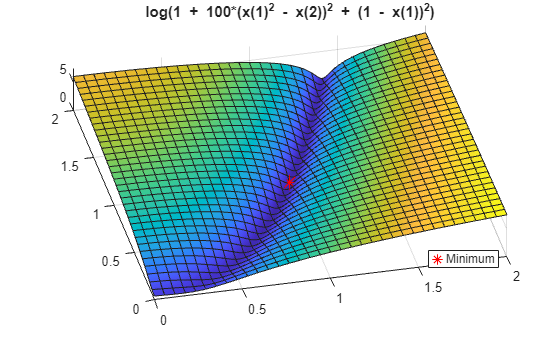# Coding and Minimizing a Fitness Function Using the Genetic Algorithm

This example shows how to create and minimize a fitness function for the genetic algorithm solver `ga` using three techniques:

• Basic

• Vectorized for speed

### Basic Fitness Function

The basic fitness function is Rosenbrock's function, a common test function for optimizers. The function is a sum of squares:

`$f\left(x\right)=100\left({x}_{1}^{2}-{x}_{2}{\right)}^{2}+\left(1-{x}_{1}{\right)}^{2}.$`

The function has a minimum value of zero at the point `[1,1]`. Because the Rosenbrock function is quite steep, plot the logarithm of one plus the function.

```fsurf(@(x,y)log(1 + 100*(x.^2 - y).^2 + (1 - x).^2),[0,2]) title('log(1 + 100*(x(1)^2 - x(2))^2 + (1 - x(1))^2)') view(-13,78) hold on h1 = plot3(1,1,0.1,'r*','MarkerSize',12); legend(h1,'Minimum','Location','best'); hold off```### Fitness Function Code

The `simple_fitness` function file implements Rosenbrock's function.

`type simple_fitness`
```function y = simple_fitness(x) %SIMPLE_FITNESS fitness function for GA % Copyright 2004 The MathWorks, Inc. y = 100 * (x(1)^2 - x(2)) ^2 + (1 - x(1))^2; ```

A fitness function must take one input `x` where `x` is a row vector with as many elements as number of variables in the problem. The fitness function computes the value of the function and returns that scalar value in its one return argument `y`.

### Minimize Using `ga`

To minimize the fitness function using `ga`, pass a function handle to the fitness function as well as the number of variables in the problem. To have `ga` examine the relevant region, include bounds `-3 <= x(i) <= 3`. Pass the bounds as the fifth and sixth arguments after `numberOfVariables`. For `ga` syntax details, see `ga`.

`ga` is a random algorithm. For reproducibility, set the random number stream.

```rng default % For reproducibility FitnessFunction = @simple_fitness; numberOfVariables = 2; lb = [-3,-3]; ub = [3,3]; [x,fval] = ga(FitnessFunction,numberOfVariables,[],[],[],[],lb,ub)```
```Optimization terminated: maximum number of generations exceeded. ```
```x = 1×2 1.5083 2.2781 ```
```fval = 0.2594 ```

The `x` returned by the solver is the best point in the final population computed by `ga`. The `fval` is the value of the function `simple_fitness` evaluated at the point `x`. `ga` did not find an especially good solution. For ways to improve the solution, see Effects of Genetic Algorithm Options.

### Fitness Function with Additional Parameters

Sometimes your fitness function has extra parameters that act as constants during the optimization. For example, a generalized Rosenbrock's function can have extra parameters representing the constants 100 and 1:

`$f\left(x,a,b\right)=a\left({x}_{1}^{2}-{x}_{2}{\right)}^{2}+\left(b-{x}_{1}{\right)}^{2}.$`

`a` and `b` are parameters to the fitness function that act as constants during the optimization (they are not varied as part of the minimization). The `parameterized_fitness.m` file implements this parameterized fitness function.

`type parameterized_fitness`
```function y = parameterized_fitness(x,p1,p2) %PARAMETERIZED_FITNESS fitness function for GA % Copyright 2004 The MathWorks, Inc. y = p1 * (x(1)^2 - x(2)) ^2 + (p2 - x(1))^2; ```

Use an anonymous function to capture the values of the additional arguments, namely, the constants `a` and `b`. Create a function handle `FitnessFunction` to an anonymous function that takes one input `x`, and calls `parameterized_fitness` with `x`, `a`, and `b`. The anonymous function contains the values of a and b that exist when the function handle is created.

```a = 100; b = 1; % define constant values FitnessFunction = @(x) parameterized_fitness(x,a,b); [x,fval] = ga(FitnessFunction,numberOfVariables,[],[],[],[],lb,ub)```
```Optimization terminated: maximum number of generations exceeded. ```
```x = 1×2 1.3198 1.7434 ```
```fval = 0.1025 ```

### Vectorized Fitness Function

To gain speed, vectorize your fitness function. A vectorized fitness function computes the fitness of a collection of points at once, which generally saves time over evaluating these points individually. To write a vectorized fitness function, have your function accept a matrix, where each matrix row represents one point, and have the fitness function return a column vector of fitness function values.

To change the `parameterized_fitness` function file to a vectorized form:

• Change each variable `x(i)` to `x(:,i)`, meaning the column vector of variables corresponding to `x(i)`.

• Change each vector multiplication `*` to `.*` and each exponentiation `^` to `.^` indicating that the operations are element-wise. There are no vector multiplications in this code, so simply change the exponents.

`type vectorized_fitness`
```function y = vectorized_fitness(x,p1,p2) %VECTORIZED_FITNESS fitness function for GA % Copyright 2004-2010 The MathWorks, Inc. y = p1 * (x(:,1).^2 - x(:,2)).^2 + (p2 - x(:,1)).^2; ```

This vectorized version of the fitness function takes a matrix `x` with an arbitrary number of points, meaning and arbitrary number of rows, and returns a column vector `y` with the same number of rows as `x`.

Tell the solver that the fitness function is vectorized in the `'UseVectorized'` option.

`options = optimoptions(@ga,'UseVectorized',true);`

Include options as the last argument to `ga`.

```VFitnessFunction = @(x) vectorized_fitness(x,100,1); [x,fval] = ga(VFitnessFunction,numberOfVariables,[],[],[],[],lb,ub,[],options)```
```Optimization terminated: maximum number of generations exceeded. ```
```x = 1×2 1.6219 2.6334 ```
```fval = 0.3876 ```

What is the difference in speed? Time the optimization both with and without vectorization.

```tic [x,fval] = ga(VFitnessFunction,numberOfVariables,[],[],[],[],lb,ub,[],options);```
```Optimization terminated: maximum number of generations exceeded. ```
```v = toc; tic [x,fval] = ga(FitnessFunction,numberOfVariables,[],[],[],[],lb,ub);```
```Optimization terminated: maximum number of generations exceeded. ```
```nv = toc; fprintf('Using vectorization took %f seconds. No vectorization took %f seconds.\n',v,nv)```
```Using vectorization took 0.153337 seconds. No vectorization took 0.212880 seconds. ```

In this case, the improvement by vectorization was not great, because computing the fitness function takes very little time. However, for more time-consuming fitness functions, vectorization can be helpful. See Vectorize the Fitness Function.

## Support평가판 신청